Newton, Chebyshev, and Halley Basins of Attraction;
A Complete Geometric Approach

Bart D. Stewart
Department of Mathematics

ab8146@exmail.usma.army.mil
BSTRACT

Real world phenomena commonly exhibit nonlinear relationships, complex geometry, and intricate processes. Analytic or exact solution methods only address a minor class of such phenomena. Consequently, numerical approximation methods, such as root-finding methods, can be used. These root-finding methods include Newton-Raphson, Chebyshev, and helleys methods.

The goal is to gain a qualitative appreciation on how various root-finding methods address many prevailing real-world concerns. The concerns include, how are suitable approximation methods determined; when do root finding methods converge; and how long for convergence?

Answers to the questions were gained through examining the basins of attraction of the root-finding methods. Different methods generate different basins of attraction. In the end, each method appears to have its own advantages and disadvantages.

INTRODUCTION

BACKGROUND

Root finding methods have been of interest for a long time. Why? Often people ask qualitative questions about real-world phenomena, and they want these questions answered. To come to an answer, one must accurately model the real world phenomena in a mathematical model, and then solve the model. In many applications, the solution involves finding a root.

Constructing models is rarely a simple process. Models come in many shapes and sizes. Some of these represent a dynamical process  a recipe for how real-world phenomena interact and change over time. How these interactions and changes occur governs the choice of model. For example, the continuous model leading to a differential equation is reasonable for certain phenomena, while difference equations in the form of a recurrence relation address phenomena occurring in discrete steps. Solutions, however, are not guaranteed in every instance.

When analytical or exact methods are applicable, sometimes formulas for solutions exist. However, these methods are restrictive, often providing insight into the behavior of only a minor class of real world phenomena. Included in this category are models that can be approximated by linear relationships, simple geometry, and low dimensionality. For a great deal of real world phenomena, that is not the norm. Real world phenomena commonly exhibit nonlinear relationships, complex geometry, and intricate processes. Consequently, exact methods can be of limited practical value (Chapra, 1988).

MOTIVATION

Where analytical or exact methods fail, numerical approximation methods often succeed. One such approximation method employs difference equations. When applied to a large though finite number of steps, difference equations are closely related to the continuous behavior of a differential equation. In fact, a continuous model, y(t), can be seen as a limit of the discrete model, yn(tn) (Figure 1.2).Figure 1.2. Approximating a Differential Equation Using a Difference Equation

Although the model approaches are different, solution methods for each share common ground. In the continuous model, solution curves may be obtained from the roots of a linear, constant-coefficient differential equations characteristic polynomial. In the discrete model, solutions come from the roots of the recurrence relations characteristic polynomial. In either case, roots can be real, imaginary, or complex. Consequently, solutions can vary greatly in their dynamical behaviors.

Numerical (Root finding) methods, however, serve as the computational tools that unveil the mysteries of such dynamical behavior. Different methods, however, may produce different results from the same initial guess. So things can get really interesting!

METHODOLOGY

From a mesh of points within the complex plane, Newton-Raphson, Chebyshev, and Halley root-finding methods numerically compute the successive approximations of some nth order complex polynomials roots. In order to better grasp the effects, the results are mapped  thus, creating a geometry of basins of attractions which are the set of starting points whose trajectories are asymptotic to a bounded region (Devaney, 1989). The geometrical differences lend to the qualitative difference amongst the root-finding methods.BEHAVIORS OF DYNAMICAL SYSTEMS

While the mathematics describing dynamic behavior may be fairly straightforward, interpreting such behavior can be difficult. In order to truly grasp it, one must familiarize oneself with the role of numerical methods and the utility of mapping their geometry.

NUMERICAL METHODS ROLE

Numerical methods approximate solutions to mathematically expressed models. When these solutions are obtained from the zeros of some functions, root-finding methods serve as the tool of choice. These methods are usually iterative  beginning with an initial starting value and computing successive approximate solutions using a well-defined recurrence relation (Figure 2.1). Each successive step yields a numerical solution to the recurrence relation  in essence, generating a sequence of even better approximations. Hence, the solution process itself is a discrete dynamical system that generates a sequence of numbers. Each term of the sequence not only signifies a numerical solution for the nth iterative step, but also suggests the ultimate behavior of that solution. Determining such behavior is not always done by simple inspection. Some sequences are obvious; others are not. Consequently, numbers alone are often not enough.

 Sample Recurrence Relation Idea of Successive ApproximationsFigure 2.1. Recurrence Relation & Iteration

UTILITY OF MAPPING  SINGLE FIXED POINT

Another, often preferred, method used to determine dynamical behaviors is to visualize them. Visualization entails mapping out the geometry of the numerical solutions. Why is this geometry important? Simply put, it graphically depicts the dynamical behavior of root-finding methods.

As Figure 2.2 suggests, the mapping of a sequence of numerical solutions depicts the behavioral path or trajectory of a single starting point. Starting points that do not change after iteration are called fixed, and qualitative behaviors of other starting points can be interpreted in relation to the fixed points.

 Sequence I Sequence II Sequence III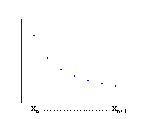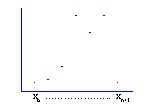Figure 2.2. Mapping of Numerical Solutions

Cobweb diagrams help point out the qualitative behaviors near fixed points using the principle of feedback (Figure 2.3) (after Peitgen, 1992). The principle of feedback is simple  an input, xn, is given, processed through some function, f, and then the output, yn, becomes the next input, xn+1, repeatedly. When allowing the ouput to equal the next input, an identity exists so that. Cobweb diagrams exploit the relationship, map the iterations, and reveal the behaviors of fixed points.Figure 2.3. From the Principle of Feedback to the Cobweb Diagram

Behaviors about fixed points are converging, diverging or chaotic, and all can be mapped. Convergent mappings point out attracting fixed points; divergent mappings denote repulsive ones; and chaotic mappings never settle. While all three behaviors are essential in describing dynamical behavior, a simple example excluding chaotic mappings will suffice to illustrate the point. Consider a simple linear recurrence relation;. When, the mapping contracts and converges to a fixed point. When, the mapping expands diverging off to positive or negative infinity. Figure 2.4 clarifies the point.Figure 2.4. Cobweb Diagrams

With relatively little effort, the geometrical approach can handle nonlinear behavior as well. For smooth nonlinear recurrence relations, the same sort of contracting and expanding argument holds near the fixed point. As Figure 2.5 indicates, the trick is to locally reduce the nonlinear model to linear parts, apply the graphical analysis, and then couple the pieces together.Figure 2.5. Concept of Linearizing a Nonlinear Mapping Function

Although dynamic behaviors about a single fixed point are fairly predictable, startling behavioral effects can and often do occur when multiple fixed points exist.

UTILITY OF MAPPING  MULTIPLE FIXED POINT

When fixed points coexist, the geometry of numerical solutions can change considerably. The effect of each fixed point is no longer simple; rather their effects interact. Consequently, determining such points and their effect is a necessity.

Multiple fixed points are often found in the realm of nonlinear phenomena. While the effect of a single fixed point has been discussed, what happens when there are two, three, or n of them? How many can there be when the iterator is a root approximation method? As Figure 2.6 points out, the fundamental theorem of algebra tells us that an nth degree polynomial is factorable into n linear factors and contains exactly n roots, which are not necessarily distinct. Whether these points are real, imaginary or complex, their coexistence may create surprising behaviors.

 Every nth-order polynomial possesses exactly n roots Any polynomial of the form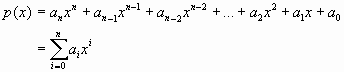can always be expressed aswhere the points zi are the polynomial roots, and they may be real, imaginary or complex. Figure 2.6. Fundamental Theorem of Algebra (after Smith, 1977)

With each additional fixed point, coexisting attractors can exhibit varying behaviors. Such behaviors are actually emerging in a sort of competitive state  with each vying to influence a solutions trajectory. As Figure 2.7 suggests, such behavioral effects may or may not extend globally. Each attracting region is called a basin of attraction  the set of starting points whose trajectories are asymptotic to a bounded region. Competition amongst the fixed points, in the effect upon xn, exists near and on basin boundaries. Moving the fixed points can create new basins and destroy old ones.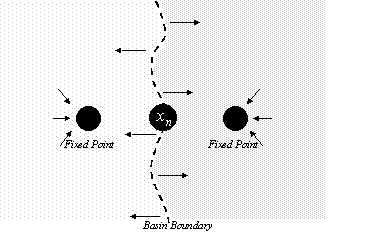Figure 2.7. Competing Effects of Multiple Fixed Points

Basin boundaries can take on infinitely many shapes. And basin boundaries can be far more complicated than a simple curve, and in most instances are. Within their intricate patterns, commonly referred to as Julia sets, is the key that reveals some erratic behaviors. Where the basins interact and compete, the behavior is not so obvious. Figure 2.8 demonstrates how nearby starting points, which are expected to have similar behaviors, can assume distinct solution paths, particularly near basin boundaries. Consider each starting point, x1, x2, and x3. Despite their nearness, starting points x1 and x3 reach a root (through different roots) while x2, which begins on a basin boundary, never settles. Hence, behaviors have a sensitive dependence on starting points, and can, at times, be considered chaotic.Figure 2.8. Behavioral Effects of Multiple Fixed Points (after Pergler, 1999)

JULIA SETS

Further consideration of such behaviors begins with observing the role of Julia sets. Julia sets are the boundary of basins of attraction -- distinguishing which starting points are prisoners to some fixed points basin, and others that escape them. Consider the following example in Figure 2.9 (after Peitgen, 1992). Note that prisoner points converge to some basin, while escapees, had any existed, would never settle. While the Julia set may be quite complicated, its role remains crucial in revealing the coexistence and competition of complex behavior.

Rules: Within the bounded region, select an arbitrary starting point. Move about to the next point as indicated (by Newton-Raphsons method for cube roots of unity), and continue until one, the path halts  in which the next destination is itself (marked by X), or two, the path becomes cyclical. Evaluate each starting point.

 L K2 K3 K3 K4 K4 I5 I6 I7 I8 I8 I9 K K3 K3 K3 I3 H4 H5 G7 H8 H9 H9 I I3 I3 K4 K3 I2 G3 F5 F7 F8 G9 G10 H H3 I4 K5 L3 K1 D2 B5 D7 F9 F9 G10 G G4 H5 K7 K6 LA A2 C7 C10 E10 F10 F10 F F4 F6 F9 F10 F11 F11 F11 F11 F11 F10 E E4 D5 B7 B6 A2 L2 I7 I10 G10 F10 F10 D D3 C4 B5 A3 B1 H2 K5 H7 F9 F9 E10 C C3 C3 B4 B3 C2 E3 F5 F7 F8 E9 E10 B B3 B3 B3 C3 D4 D5 E7 D8 D9 D9 A B2 B3 B3 B4 B4 C5 C6 C7 C8 C8 C9 1 2 3 4 5 6 7 8 9 10 11

While it is apparent that three basins of attraction exist, there is another valuable piece of information. Through adding the number of moves necessary from an arbitrary starting point to a fixed point and coloring the basins of attraction, the Julia set (approximated by the white boundary) becomes apparent.

 2 1 1 2 2 5 6 4 4 4 4 1 1 1 3 3 3 5 6 4 4 3 3 2 1 4 5 3 3 3 3 2 5 2 4 2 2 3 4 5 3 3 2 5 3 4 4 2 2 4 4 2 1 1 2 3 3 1 2 2 2 2 2 1 5 3 4 4 2 2 4 4 2 1 1 5 2 4 2 2 3 4 5 3 3 2 3 3 2 1 4 5 3 3 3 3 2 1 1 1 3 3 3 5 6 4 4 2 1 1 2 2 5 6 4 4 4 4
Figure 2.9. Julia Set Example

NUMERICAL METHODS

Since numerical methods are capable of approximating the zeros of an analytic function, root-finding methods serve as the tool of choice. Such methods come in many shapes and sizes. Some are rather simple; others are complex. Each, however, employs a different iterative approach that affects the geometry of numerical solutions, and thus impacts on dynamical interpretations. Consequently, an investigation of such methods is necessary.

Although there are many root-finding methods to choose from, their conceptual origin is the same  that is, all stem from a successive point-wise approximation of an arbitrary functions root. For example, Taylors theorem approximates a function value and, when truncated accordingly, is a numerical method of some sort. Figure 3.1 illustrates another, and more recent, method yielding a one-parameter family, e, of single-point numerical methods capable of finding roots (after Popovski, 1979). Of particular interest were the Newton-Raphson, Chebyshev, and Halley methods.

For illustrative purposes, a uniform approach is applied. No matter the root-finding method, each considers the function, f(z)= z3-1, and is restricted to real arithmetic for its geometrical interpretation. From an arbitrary point, xo, approximations are computed so as to satisfy Popovskis single-point method,. Successive computations yield more approximations, x0, x1, x2,, xn. To ensure the dynamic behavior is clear, approximations are represented numerically and geometrically.

 Solving f(z)=0 can be found on the basis of a single-point approximation by the four parameter functionConsider a function f(z) on an interval [a,b] where f(a)f(b)<0 and f(z)f"(z)¹ 0, zÎ [a,b]. Let ziÎ[a,b] be the ith approximation to the root rÎ [a,b] of f(z)=0. Then the following approximation to the root r, zi+1 may be obtained from the system of equations whereand when solved yields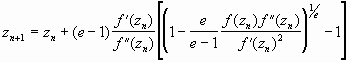Figure 3.1. Popovskis Single-Point Iteration Formula

No matter the function to be approximated, special parameter values, e, reveal familiar methods. When e approaches one, the single point iteration formula reduces to the popular Newton-Raphson method (Figure 3.2). The approximation method simply computes a tangent line to the point xn of our function. When e equals one-half, the single point iteration formula reduces to Chebyshevs method (Figure 3.3). The approximation method, rather than line, computes a tangent horizontal parabola to the point xn of our function. When e equals negative one, the single point iteration formula reduces to Halleys method (Figure 3.4). The approximation method computes a tangent hyperbola to the point xn of our function. For each of these approximations, the root of its tangent typically represents a better approximation to our functions root.Figure 3.2. Newton-Raphson Method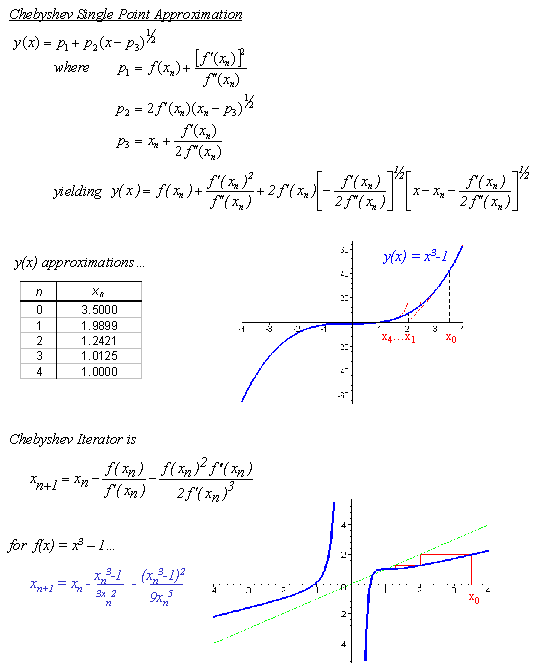Figure 3.3. Chebyshev MethodFigure 3.4. Halley Method

While each method can determine the appropriate root, certain methods are preferred. As Figure 3.6 illustrates, when monotonic behavior exists, the preferential order is clear  Halley, Chebyshev, and then Newton-Raphson. In Halley and Chebyshev, the approximating curves echo the shape of our function. Newton-Raphsons approximating curve is restricted to a simple line. While in this instance convergence is guaranteed, it varies according to the step sizes of the approximating methods. With the smallest step size, Newton-Raphson is only quadratically convergent while the other methods having larger step sizes are cubically convergent. When a function is not monotonic, the preference is generally uncertain  with no single method consistently better than the others. Clues to determining such an ordering begins with the careful observation of each methods geometry.numerical Methods geometry

Again, numerical methods can sort through a dynamical systems behavior through finding its roots and examining their affect. With different methods yielding different behaviors, an examination of individual numerical methods is necessary. Recall how our three numerical methods, while obtaining the appropriate root, all sought distinct solution paths to it. Consider now a mesh of complex starting points, rather than a single real point, with an assortment of fixed points. What are the numerical solution paths now? What is the effect of the competition and coexistence of fixed points? What numerical method is preferred, if any? Answers to these questions appear when mapping and coloring each numerical methods solutions.

While an nth order complex polynomial with distinct roots partitions the complex plane into n number of basins, the partitions may or may not be equally distributed  or even connected for that matter. In an ideal setting, these attracting regions resemble a Voronoi diagram  regions containing all points that are the nearest neighbors to the polynomials zero (Figure 4.1). Few things, though, are ideal. Rather, an attracting region contains all starting points that asymptotically approach the zero, despite their locality.

 Single Fixed Point Multiple Fixed Points Ideal Basins (Voronoi Diagrams) Calculated BasinsFigure 4.1. Basins of Attraction

One popular method to visualize these regions is basin coloring. The process simply assigns n colors to the n basins, executes some numerical method to calculate which initial points within a bounded region or mesh converge to a particular basin, and paints that basins color to that point (Figure 4.2). Furthermore, the number of iterations necessary to converge to a root can be shown through variety of color intensities. Points calling for fewer iterations appear with greater intensity. Through employing these tools, sensible geometric interpretations are possible for nearly all complex polynomials.

 Single Fixed Point Multiple Fixed Points Ideal Basins (Voronoi Diagrams) Calculated Basins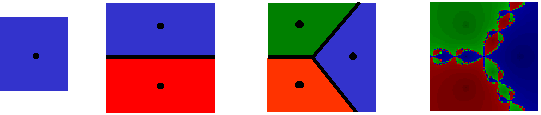Figure 4.2. Basins of Attraction Coloring

PURE REAL AND PURE IMAGINARY ROOTS

When considering pure real or pure imaginary roots, the geometries, while still creating a variety of basin shapes, sizes, and complexities, are remarkably similar  only differing by a rotation to the appropriate coordinate the axes. Consequently, observations for one case support the other.

Even in what appears to be the simplest of settings, real roots, our basins of attraction are not ideal (Figure 4.3). Whether considering the case of equally or unequally distributed roots (Figures 4.4 & 4.5), nearest neighbor convergence fails to hold. The shape of the basins roughly appears to conform to that of a hyperbola. Symmetry is another common factor that plays a role in shaping the basins. Equally distributed roots generate symmetry throughout the geometry; unequally distributed roots do not.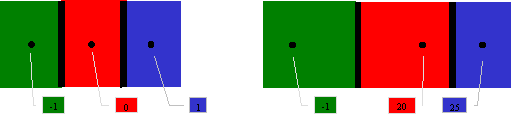Figure 4.3. Ideal Basins & Associated Roots

With slight exceptions along basin boundaries, the basin sizes for these are fairly comparable. For each, there exists some effective radius of convergence  that is, points in the neighborhood of a root tend toward that particular root (Figure 4.4). As Figures 4.4 suggests, that effective convergence radius not only changes amongst the numerical methods applied, but also with each polynomial considered. With higher order polynomials commonly creating more and more complex geometries, such radii are often greatly reduced.

Each method also bears some sensitive dependence on starting conditions. With nearby starting points assuming distinct solution paths, unpredictable behaviors can result. Although Figure 2.8 pointed out the concept initially, chaos impact cannot be ignored  particularly with it present in every methods geometry. Figure 4.6 further reveals that basin boundaries may be self-similar, with infinite levels of detail, characteristic of fractal geometry.

 Newton-Raphson Chebyshev Halley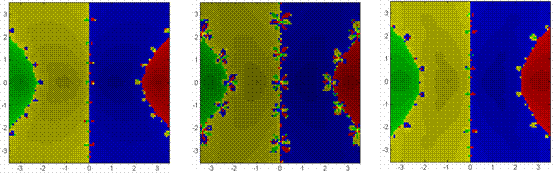Figure 4.4. 3rd, 4th, & 5th Order Equally Spaced Roots

 Newton-Raphson Chebyshev Halley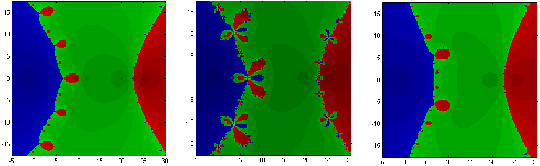Figure 4.5. 3rd, 4th, & 5th Order Unequally Spaced Roots

 Newton-Raphson Chebyshev HalleyFigure 4.6. Chaos Everywhere

In considering the sensitive dependence on starting conditions, one need to only observe the decorations along the basin boundaries for each methods geometry in terms frequency, size, and structure. As a consequence of the competition and coexistence of more and more fixed points, the decorations appear with greater frequency with higher order polynomials, yet their size decreases. Whether equally or unequally spaced real roots, the Newton-Raphson, Chebyshev and Halley methods appear to experience chaotic dynamics in a phase-shifted manner with each other  an unexpected outcome of their iterators.

While pure roots create nifty geometries, things get really interesting with mixed roots.

MIXED ROOTS

Mixed roots, those roots containing both real and imaginary components, provide a rich variety of basin shapes, sizes, and complexities. In many instances, there are striking similarities amongst these types of roots and pure ones. But when differences appear, a spectrum of spectacular geometries develops.

ROOTS OF UNITY (EQUALLY DISTRIBUTED ROOTS)

In the simplest of settings, roots of unity, there are more similarities than differences. Nearest neighbor convergence fails to hold. As expected from the equal distribution of roots, basin shapes are symmetric (Figures 4.7). Again, these shapes lend to the equally distributed sizes of each basin.

Basin boundaries vary considerably  spanning from the very simple to the exceptionally intricate. Consequently, basins are more disconnected. As for sensitive dependence on starting conditions, the decorations for each methods geometry in terms frequency, size, and structure remains similar to the case of real roots. Furthermore, the effective radius of convergence is also affected accordingly (Figure 4.7). Figures 4.7 & 4.8 suggest, that effective convergence radius not only changes amongst the numerical methods applied, but also with each polynomial considered.

Again, as larger order polynomials are considered, basins become more complicated. Such behavior occurred previously, so this is of no great surprise.

 Newton-Raphson Chebyshev Halley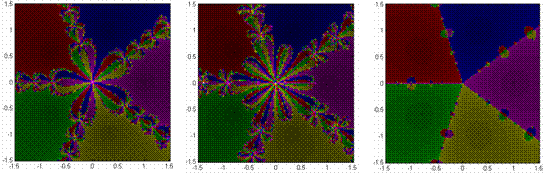Figure 4.7. 3rd & 5th Order Equally Spaced Roots (of Unity)

UNEQUALLY DISTRIBUTED ROOTS

When roots are positioned irregularly, interesting things can and do happen. In general, the concepts of nearest neighbor convergence failing, basin shape, size, and complexity are as noted previously  but perhaps in a more pronounced fashion.

In general, these third order polynomials can produce a variety of familiar behaviors  echoing those previously found with real roots. In certain instances, one is nothing more than a skewed version of the other. When one fixed point is a near-enough reflection of another, a near-enough symmetric geometry results; otherwise, a distorted geometry develops. Preference for a particular numerical method is subject to the presence of such symmetry.

Figure 4.8 reveals how convergence changes with the higher order polynomials  with many containing irregular and unexpected basins shapes and decorations as a result of the competition and coexistence of additional fixed points. Halleys method generates the most pronounced irregularities, to include distinct breaks in basin connectivity.

In all these instances, basin shapes, sizes and complexities vary considerably. And in nearly every case, the geometry remains unpredictable. But within this chaotic environment, is there any order?

 Newton-Raphson Chebyshev Halley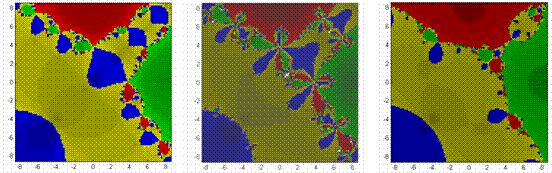Figure 4.8. Various Orders of Unequally Spaced Roots

CONCLUSIONS AND RNDATIONS

CONCLUSIONS

The Newton-Raphson, Chebyshev, and Halley approximation methods, serve as powerful tools in evaluating complex polynomials roots. These different methods, however, can yield different solutions from identical starting points. In determining any preference for the numerical methods, consideration must be given to the polynomial at hand, when do root finding methods converge and how long for convergence.

In many instances, the Newton-Raphson and Halley geometries are nearly indistinguishable. When fixed points are a reflection of another, Halleys method assumes a much larger effective radius over the Newton-Raphson method. Chebyshevs method, filled with complex boundaries and relatively small effective radii, remains the worst of the three.

With the methods relatively comparable in computational speed, the greater emphasis rests in basin shape, size, and complexity.

Ultimately though, the method of choice depends on the complex polynomial at hand.

RECOMMENDATIONS

While room for further research in this topic exists, a particular effort with respect to more numerical methods, calculating effective radii, and consideration for repeated roots would prove both challenging and rewarding.

REFERENCES

Chapra, S.C. and Canale, R.P., Numerical Methods for Engineers, 2d Ed., McGraw-Hill Book Company, 1988.

Devaney, Rober L., An Introduction to Chaotic Dynamical Systems, 2d Ed., Addison-Wesley, 1989.

Hansen, E. and Patrick, M., "A Family of Root Finding Methods," Numerische Mathematik, v. 27, pp. 257-269, 10 February 1976.

Melman, A., "Geometry and Convergence of Eulers and Halleys Methods," SIAM Review, v. 39, pp. 728-735, December 1997.

Peitgen, H., Jurgens, H., and Saupe, D., Chaos and Fractals: New Frontiers of Science, Springer, 1992.

Pergler, Martin, "Newton's method and Newton basin fractals." [http://www.math.uchicago.edu/~pergler/genteach/newton/newton.html]. November 1999.

Popovski, D.B., "A Family of One-Point Iteration Formulae for Finding Roots," International Journal of Computation Mathematics Section B, v. 8 pp. 85-88, July 1979.

Press, W.H., and others, Numerical Recipes in C: The Art of Scientific Computing, pp. 279-280, Cambridge University Press, 1988.

Smith, Julius O., "The Fundamental Theorem of Algebra." [http://ccrma-www.stanford.edu/~jos/complex/Fundamental_Theorem_Algebra.html]. April 2001.

Strogatz, Steven H., Nonlinear Dynamics and Chaos, Perseus Books, 1994.

VisMathHOME